Mathematics
Easy

Question

#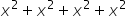##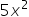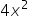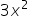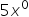Hint:

## The correct answer is:### The given expression is x2 + x2 + x2+ x2There is one variable in above expression. The variable is: ‘x’. There are four terms in the above expression. We have to perform the operations between them and find the right answer.If we see all the terms has x raised to power two. Such terms with the same variables or same variable raised to same powers are called “like terms.” And the terms with different variables are called “unlike terms.”For like terms, we can perform the operations between their coefficients by keeping the variable constant. It means we can treat each term as a constant and perform operations like normal operations on constants.For the above expression we will keep x square as constant as it is common variable. We will add the coefficients of the terms.Let’s see the above expressionx2+ x2 + x2+ x2 = ( 1 + 1 + 1 + 1 ) x2= 4x2Therefore, the value of the equation is 4x2So option which is ’4x2’, is the right option.

For terms to be like terms the power should be same too. Only like terms are operated with each other.

### Related Questions to study#### With Turito Foundation.#### Get an Expert Advice From Turito.